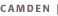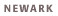# Graduate Courses in Mathematics for Teachers (644)16:644:501 (S) Seminar in Mathematical Ideas (3) Problematical yet fundamental ideas in the mathematics curriculum; explorations of the historical development; logical and cognitive issues involved in understanding and applying those ideas. Goldin. Prerequisites: Permission of the instructor; not open to degree candidates in mathematics (640,642); ordinarily restricted to experienced K-8 teachers and to graduate students in mathematics education. 16:644:502 (Su) Transition from Arithmetic to Algebra for Middle School Teachers (3) Properties of basic number systems (counting, numbers, integers, rationals, reals). Algebraic expressions. Equations and inequalities; their meaning, solution, applications, quantifiers. Coordinate systems. Functions and their graphs. Emphasis on reasoning and explanation. Beals, Lyons, Wilson. Prerequisites: Permission of the instructor; not open to degree candidates in mathematics (640,642); ordinarily restricted to experienced K-8 teachers and to graduate students in mathematics education. 16:644:503 (Su) Geometry and Measurement for Middle School Teachers (3) Basic properties and measurement of geometric objects. Rigid motions and symmetry, similarity. Perimeter, area, and volume for polygons and polyhedrons. Approximating these quantities for curvilinear objects. Explanation and justification. Cohen-Corwin, Woodward.  Prerequisites: Permission of the instructor; not open to degree candidates in mathematics (640,642); ordinarily restricted to experienced K-8 teachers and to graduate students in mathematics education. 16:644:504 (Su) Problem Solving with Discrete Mathematics for the Middle Grades (3) Elementary mathematical modeling and problem solving arising from discrete mathematics, systematic counting, map coloring, use of vertex-and-edge graphs, and number patterns. Rosenstein. Prerequisites: Permission of the instructor; not open to degree candidates in mathematics (640,642); ordinarily restricted to experienced K-8 teachers and to graduate students in mathematics education. 16:644:505 (Su) Data and Probability for Middle School Teachers (3) Display and analysis of data (median, mean, variance, stem-and-leaf plots; box-and-whisker plots); empirical and theoretical approaches to finite probability and uniform distributions. Risk. Prerequisites: Permission of the instructor; not open to degree candidates in mathematics (640,642); ordinarily restricted to experienced K-8 teachers and to graduate students in mathematics education.